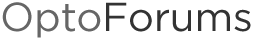# 4-20mA Conversion Formula

I have a sensor which gives me a raw input of the range 4000-20000. This represents a scaled range of 0-1450. Can anyone give me a formula that will allow me to calculate the scaled value from the raw one.

I’ll make up an example: I get a raw reading of 12456. How do I convert that to a scaled value? I know you can do it in Groov with the scaling tool but I need to do it as a formula.

Thanks,

Dave

Dave,

You have an elevated Zero (4000 = 0 Units) so you need to subtract that from the 12456 to start with. The span of your sensor 0-1450 units = 16000 (20000-4000).
Once the zero is taken care of it’s a simple percentage or ratio calculation.

((Raw Reading - 4000)/16000) x 1450 = Value

(12456 - 4000) = 8456

8456 / 16000 = 0.5285 i.e your reading is 52.85% of the 1450

0.5285 x 1450 = 766.325 units

another way to visualize it.

0% = 4000
25% = 8000
50% = 12000
75% = 16000
100% = 20000

To go from units to the raw value would be the same except you’d need to add the zero (4000) to the ratio.

What would raw value of 725 units be?

((725/1450) x 16000) + 4000

725/1450 = 0.5
0.5 x 16000 = 8000
8000 + 4000 = 12000

2 Likes

Thanks! That’s perfect.

I probably should have paid more attention in math class. :-}

Dave

1 Like